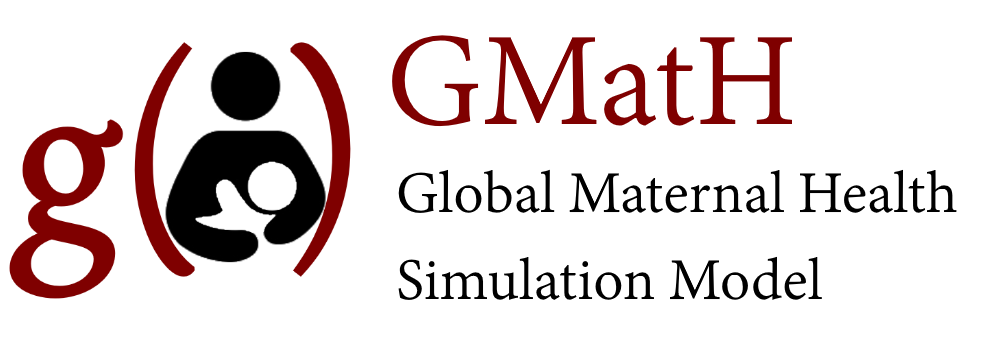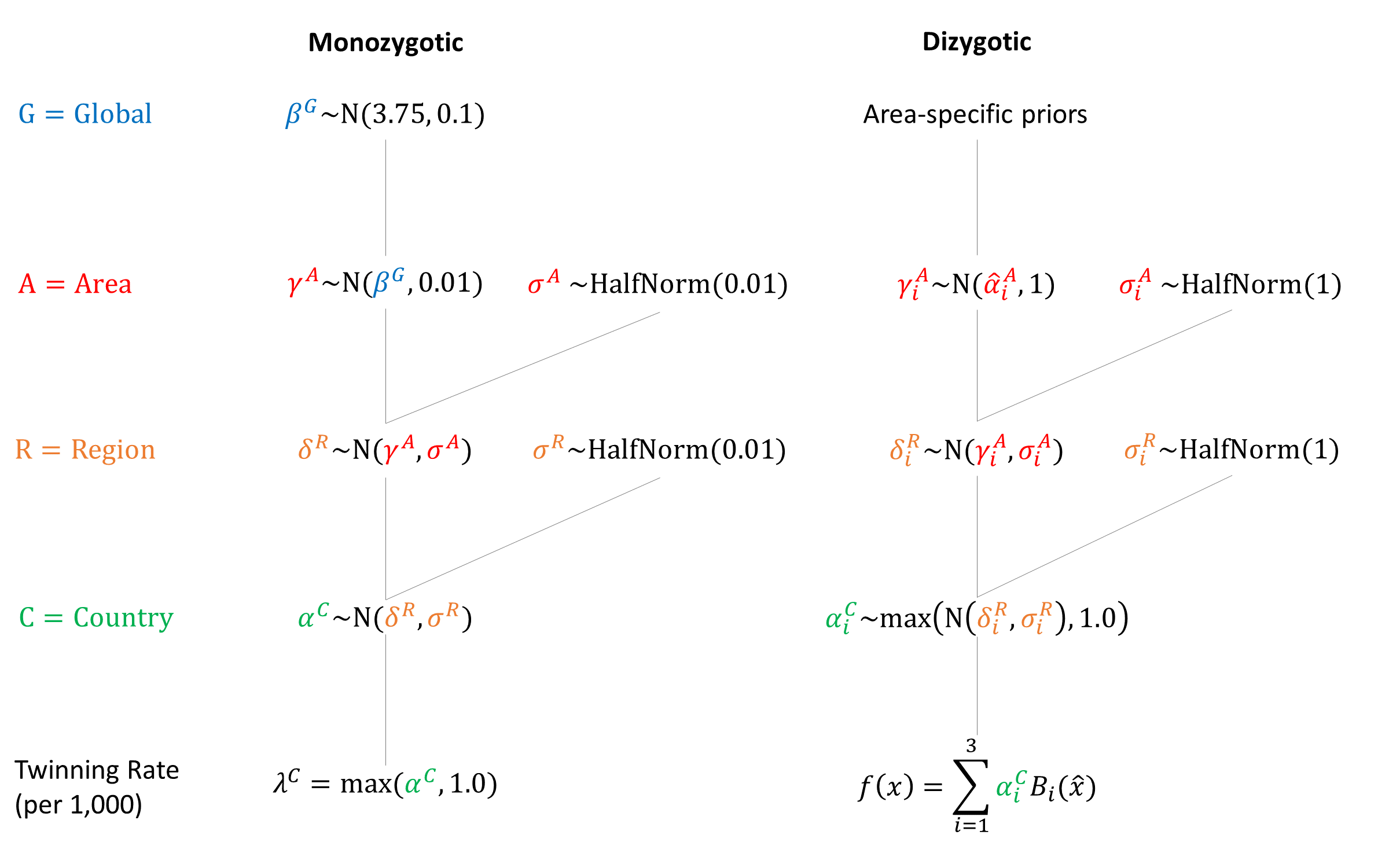Model Inputs $$\rightarrow$$ Biological Parameters $$\rightarrow$$ Twinning Rates

### Overview

Twins are simulated in the model to more accurately represent the number of births in the population, and to account for increased risks of delivery complications that may occur with multiple fetuses. We do not model triplets or higher order multiples due to their very low incidence.

### Data

Monozygotic twinning is thought to occur at a relatively constant rate of 3.5–4 per 1000 births across human populations.[2,3] However, the rate of dizygotic twinning varies greatly around the world, leading to large differences in overall twinning rates, which range from about 6 per 1000 births in Asia, to 10-20 per 1000 births in Europe and the USA, to about 40 per 1000 births in parts of Africa.

A study based on 150 Demographic and Health Surveys, conducted between 1987 and 2010 in 75 low and middle income countries found similar differences in twinning rates, with variation caused by dizygotic twinning rates. In developed countries, twinning rates have been increasing. A study of trends in the US found that only about 1/3 of the increase was due to increasing maternal age, with the rest likely explained by increased use of infertility treatments.

The number of twin pregnancies increases substantially with maternal age,[3,6] with estimated twinning rates increasing more than 4-fold between the ages 15 and 37 years with parity held constant.[7,8]

Several studies have reported twinning rates (per 1,000) by maternal age:

Maternal Age Monozygotic Dizygotic Country Source
<20 5.4 3.6 Israel Harlap 1979
20-24 5.1 5.8
25-29 5.0 11.3
30-34 5.1 8.3
35-39 7.3 17.3
$$\geq$$ 40 7.4
$$\leq$$ 29 4.2 32.3 Nigeria Nylander 1981
$$\geq$$ 30 4.7 72.8
<25 3.7 1.9 India Rao 1983
25-29 4.6 5.9
30-34 1.1 10.7
$$\geq$$ 35 1.0 12.0
<19 3.6 2.5 Italy Parazzini 1991
20-24 3.6 4.2
25-29 4.2 5.4
30-34 4.1 7.3
35-39 4.5 8.1
40-44 3.8 6.0
$$\geq$$ 45 4.7
<20 0.0 2.26 India Satija 2008
20-24 3.98 3.98
25-29 3.19 11.46
30-34 2.51 20.1
<15 3.2 0.3 Brazil Otta 2016
15-19 3.6 2.1
20-24 3.9 4.2
25-29 4.7 6.6
30-34 4.9 10.1
35-39 5.0 13.0
40-44 4.2 12.5
45+ 9.1 35.1

### Parameters

We assumed that monozygotic twin rates are constant by maternal age. To model age-related dizygotic rates we used quadratic B-splines fit to the midpoint of the maternal age groups. The estimated dizygotic twinning rate at age 38 was used as the value for later maternal ages.

We set area-specific dizygotic priors for ‘low’ and ‘high’ twinning countries.

Spline Width: 30

Spline # Knot Location Africa Asia Europe Latin America North America Oceania
1 20 $$N(10,5)$$ $$N(2.5,1)$$ $$N(10,5)$$ $$N(2.5,1)$$ $$N(10,5)$$ $$N(10,5)$$
2 30 $$N(25,5)$$ $$N(10,3)$$ $$N(25,5)$$ $$N(10,3)$$ $$N(25,5)$$ $$N(25,5)$$
3 40 $$N(40,5)$$ $$N(20,3)$$ $$N(40,5)$$ $$N(20,3)$$ $$N(40,5)$$ $$N(40,5)$$### Model Implementation

At conception, the model simulates whether the pregnancy is a singleton or twins. If monozygotic twins are simulated, then a single sex is simulated based on the sex ratio and applied to both fetuses. If dizygotic twins are simulated then a sex is simulated for each fetus. We assume that sex-selective abortion does not apply to twins. Country-specific twinning rate estimates are included as calibration targets to help fit the model.GMatH (Global Maternal Health) Model - Last updated: 28 November 2022